## Why and when to use table calculations in Tableau

by Anna Noble

In Tableau you can manipulate, edit, add context, and create new fields in your data using calculated fields. All without having to go back and edit your original database. Which is pretty awesome, right?  But what if you want to do calculations based the fields you are using to create the visualisation instead of the whole data set? That’s where table calculations come in.  They allow you to use the dimensions in the view to partition the calculation you are carrying out.

Let’s start by making a calculated field and then show how we can use table calculations on our results.

## Using a calculated field to put sales in context

For example you could make a calculated field that shows the profit ratio.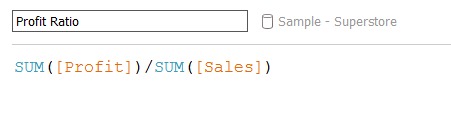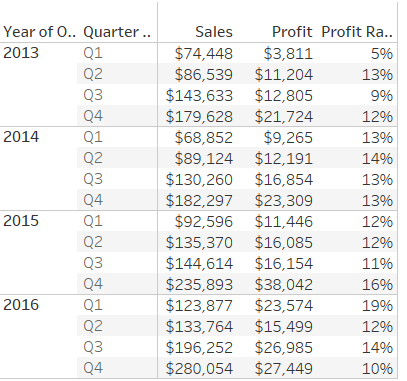But if you want to look at how the profit ratio changes in each quarter over a number of years, then you will need to use a table calculation.

## Use a Table Calculation to show how Profit Ratio changes form one quarter to the next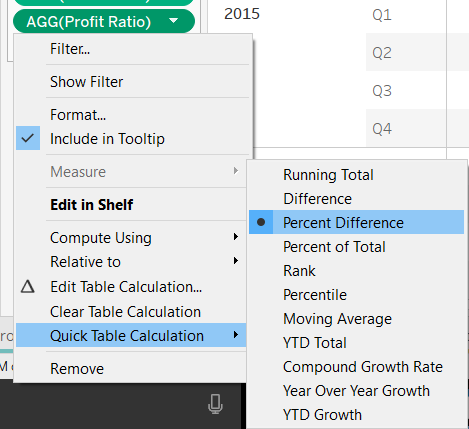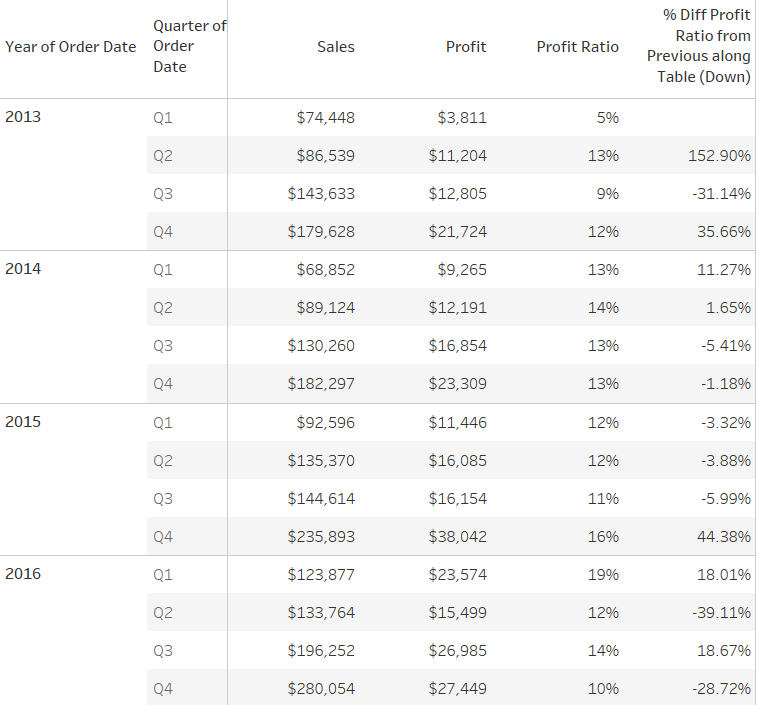If you right click on the profit ratio field within the view, then you can select quick table calculation and choose percentage difference and your percentages will change to reflect the change from the previous quarter.  Or at least that is what it looks like.

## Make sure Tableau is doing what you want

We can check the calculation Tableau is doing by right clicking and selecting edit table calculation from the drop down menu.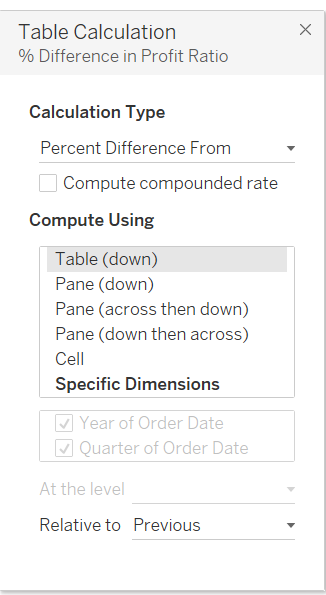You can see that the relative to is set to ‘Previous’ there are also options to use Next, First and Last within the drop down menu.  So we could look at the percentage change on profit ratio relative to the first quarter for all quarters there after.  In this case, the first quarter acts as a baseline comparison.

The result of our table calculation is also determined by which option we choose in the Compute Using function, but I’m going to let Megan walk you through that.

## So how do they work?

Table calculations use the data that’s cached in Tableau, i.e., Tableau takes the results of our calculated field to perform a new calculation that compares the profit ratio in the current quarter to the previous quarter’s results.Anna Noble

Sun 04 Mar 2018

Thu 01 Mar 2018

Tue 27 Feb 2018

Mon 06 Feb 2017